American Journal of Physical Chemistry
Volume 5, Issue 5, October 2016, Pages: 87-93

Kinetics and Mechanistic Approach to the Oxidation of Inositol by Cerium(IV) in Aqueous Perchlorate Medium

Ismail Althagafi1, *, Ahmed Fawzy1, 2, Ishaq A. Zaafarany1, Fahd A. Tirkistani1, Khalid S. Khairou1

1Chemistry Department, Faculty of Applied Science, Umm Al-Qura University, Makkah, Saudi Arabia

2Chemistry Department, Faculty of Science, Assiut University, Assiut, Egypt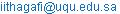(I. Althagafi)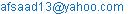(A. Fawzy)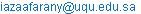(I. A. Zaafarany)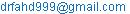(F.A. Tirkistani)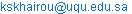(K.S. Khairou)

*Corresponding author

Ismail Althagafi, Ahmed Fawzy, Ishaq A. Zaafarany, Fahd A. Tirkistani, Khalid S. Khairou. Kinetics and Mechanistic Approach to the Oxidation of Inositol by Cerium (IV) in Aqueous Perchlorate Medium.American Journal of Physical Chemistry. Vol. 5, No. 5, 2016, pp. 87-93. doi: 10.11648/j.ajpc.20160505.11

Received: September 7, 2016; Accepted: September 23, 2016; Published: October 11, 2016

Abstract: Oxidation kinetics of inositol (INOS) by cerium(IV) has been studied spectrophotometrically in perchlorate medium at fixed ionic strength and temperature. The reaction showed a first order dependence with respect to [Ce(IV)] and less than unit order kinetics in both [H+] and [INOS]. Increasing both ionic strength and dielectric constant of the reaction medium increased the oxidation rate. Addition of Ce(III) product had no any significant effect on the oxidation rate. The oxidation product of inositol was identified by both spectroscopic and chemical tools as inosose. A plausible mechanistic scheme for oxidation reaction has been proposed and the rate law associated with the reaction mechanism was derived. The activation parameters of the second order rate constant were calculated and discussed.

Keywords: Inositol, Kinetics, Mechanism, Oxidation, Cerium(IV)

1. Introduction

Inositol is a six membered cyclic polyol, which is synthesized in the kidneys . Inositol and some of its derivatives used as the basis for some signaling and secondary messenger molecules [2,3] which are involved in insulin signal transduction, gene expression, breakdown of fats and reducing blood cholesterol . Kinetics of oxidation of inositol was investigated by different oxidants like alkaline potassium periodate , alkaline diperiodatocuprate(III) , alkalinepermanganate , vanadium(V) in acid medium  and by chromic acid .

Cerium(IV) is a significant single electron oxidant in acid media [10-23]. In perchlorate medium, this oxidant has rarely been employed [21,22], probably due to presence of dimers and polymers of cerium (IV) in such medium . Oxidation reactions by cerium(IV) in perchloric acid solutions proceed much faster than those in sulfuric acid ones.

Although a number of investigations on the kinetics of oxidation of inositol by various oxidants has been performed [5-9], there is a lack of literature on the kinetics of oxidation of this polyol compound by cerium(IV) in acidic medium. This observation prompted us to investigate the title reaction. The objectives of the present study are to check the reactivity of inositol towards cerium(IV) in perchlorate medium, to understand the active species of the reactants in such medium, and finally to propose the oxidation reaction mechanism.

2. Experimental

2.1. Materials

All chemicals used in this work were of reagent grade and their solutions were prepared by dissolving the required amounts of the samples in doubly distilled water. The stock solution of INOS was prepared by dissolving an appropriate amount of recrystallized sample (Merck) in double distilled water. A fresh solution of cerium(IV) was prepared by dissolving ceric ammonium sulfate (Sigma) in sulfuric acid, diluted with double distilled water and kept for overnight. The solution of cerium(IV) was stored in a dark glass bottle and was used after 24 h . Cerium(III) solution was also  prepared by dissolving cerium(III) acetate (Sigma) in double distilled water.

2.2. Kinetic Measurements

The kinetic measurements were followed under pseudo-first order conditions where an excess of inositol over cerium (IV) was used. The course of the reaction was monitored by following the decrease in absorbance of cerium(IV) as a function of time at λ = 316 nm, its absorption maximum, whereas the other constituents of the reaction mixtures had no significant absorption at this wavelength as shown in Fig. 1. The absorbance measurements were made in a Shimadzu UV-VIS-NIR-3600 double-beam spectrophotometer. The pseudo-first order rate constant values (kobs) were calculated as the slopes of ln (absorbance) versus time plots. The rate constants were the mean values of at least three kinetic measurements. The rate constants were reproducible to 3-4%. The spectral changes for the oxidation of inositol by cerium (IV) in perchlorate medium are shown in Fig. 1. The scanned spectra indicate gradual decay of Ce(IV) band at its absorption maximum with time as a result of its reduction to Ce(III) by inositol reductant. The reaction orders with respect to the reactants were determined from the slopes of log kobs versus log (Conc.) plots by varying the concentrations of inositol substrate and perchloric acid, in turn, while keeping other conditions constant.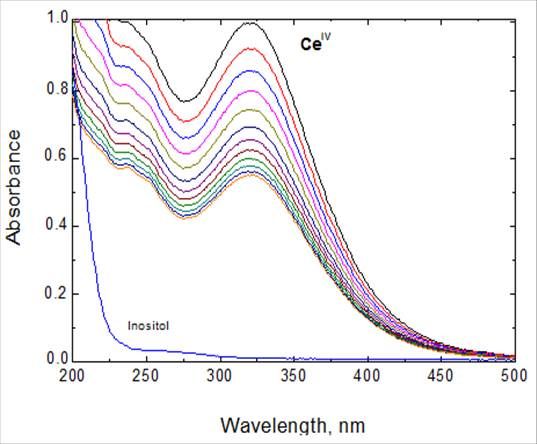Figure 1. Spectral changes during the oxidation of inositol by Ce(IV) in perchlorate medium. [INOS] = 5.0 x 10-3, [Ce(IV)] = 2.0 x 10-4, [H+] = 0.5 and I = 1.0 mol dm-3 at 25 oC. Scanning time intervals = 3 min.

3. Results

3.1. Reaction Stoichiometry and Product Analysis

Reaction mixtures containing various amounts of Ce(IV) and inositol at constant [H+], ionic strength and temperature were allowed to react for 24 h for completion of the oxidation reaction. The unconsumed [Ce(IV)] was determined spectrophotometrically at 316 nm. The results indicated that two moles of Ce(IV) are consumed by one mole of inositol to yield the final oxidation products as shown in the following equation,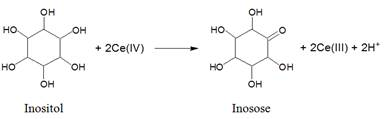(1)

The above stoichiometric equation is consistent with the results of products analysis which carried out by both spectroscopic and chemical tools [25-27]. The oxidation product of inositol was identified as the corresponding monoketone derivative, namely inosose which was also tested by addition of 2,4-dinitrophenylhydrazine to the reaction mixture .

3.2. Rate Dependence on [Ce (IV)]

The observed first order rate constant (kobs) was determined at different initial concentrations of Ce(IV) keeping other variables constant. It has been observed that the increase in the oxidant concentration does not change the oxidation rate of inositol (table 1). This indicate that the oxidation rate constant is independent of oxidant concentration and the order of reaction with respect to the oxidant is confirmed to be one.

3.3. Rate Dependence on [INOS]

The reductant inositol was varied in the range of 2.0 – 10.0 x 10-3mol dm-3 while other variables were kept constant. It was observed that increasing inositol concentration increases the oxidation rate as listed in table 1. A plot of kobs versus [INOS] was found to be linear with a positive intercept (figure 2) confirming the less than unit order dependence with respect to [INOS].

3.4. Rate Dependence on [H+]

The effect of hydrogen ion concentration on the oxidation rate was studied by varying the perchloric acid concentration in the range of (0.1 – 0.9 mol dm–3) and keeping the concentrations of all other reactants constant. It was observed that the oxidation rate increases with increasing [H+] (table 1). A plot of log kobs versus log [H+] was linear with a slope of 0.79 as shown in figure 3 confirming the fractional-first order dependence in [H+].

3.5. Effect of Ionic Strength and Dielectric Constant

The effect of ionic strength of the reaction medium on the oxidation rate was studied by varying the ionic strength in the range 1.0 – 3.0 mol dm–3 using sodium perchlorate as an inert electrolyte keeping the concentrations of all other reactants constant. The results indicated that increasing ionic strength of the medium increased the oxidation rate and the Debye–Huckel plot was linear with a positive slope of 0.94 (Figure 4). Furthermore, the effect dielectric constant (D) was also studied by varying the acetic acid - water content in the reaction mixture with all other conditions being kept constant. The rate constant clearly decreased as D of the solvent decreased (i.e., increasing acetic acid content) and the plot of log kobs versus 1/D was linear with a negative slope of -29.41 (Figure 5).

Table1. Effect of variation of [Ce(IV)], [INOS], [H+] and ionic strength (I) on the first order rate constant value, kobs, in the oxidation of inositol by Ce(IV) in perchlorate medium at 25 oC.

 104 [Ce (IV)] (mol dm-3) 103 [INOS] (mol dm-3) [H+] (mol dm-3) I (mol dm-3) 105kobs (s-1) 0.5 6.0 0.5 1.0 42.3 1.0 6.0 0.5 1.0 41.9 2.0 6.0 0.5 1.0 42.8 3.0 6.0 0.5 1.0 43.1 4.0 6.0 0.5 1.0 43.4 2.0 2.0 0.5 1.0 19.2 2.0 4.0 0.5 1.0 33.0 2.0 6.0 0.5 1.0 42.8 2.0 8.0 0.5 1.0 56.6 2.0 10.0 0.5 1.0 71.4 2.0 6.0 0.1 1.0 13.2 2.0 6.0 0.3 1.0 32.6 2.0 6.0 0.5 1.0 42.8 2.0 6.0 0.7 1.0 67.4 2.0 6.0 0.9 1.0 86.0 2.0 6.0 0.5 1.0 42.8 2.0 6.0 0.5 1.5 44.7 2.0 6.0 0.5 2.0 47.0 2.0 6.0 0.5 2.5 50.2 2.0 6.0 0.5 3.0 52.4

Experimental error ± 3%.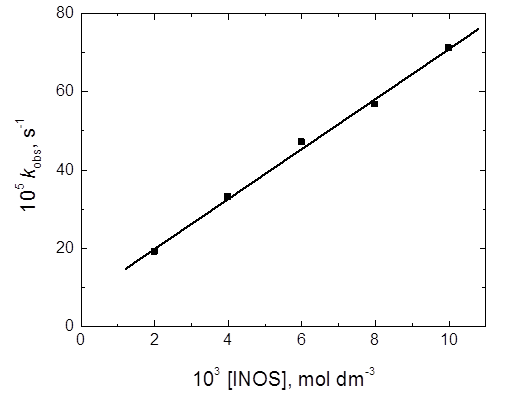Figure 2. A plot of kobs versus [INOS] in the oxidation of inositol by Ce(IV) in perchlorate medium. [Ce(IV)] = 2.0 x 10-4, [H+] = 0.5 and I = 1.0 mol dm-3 at 25 oC.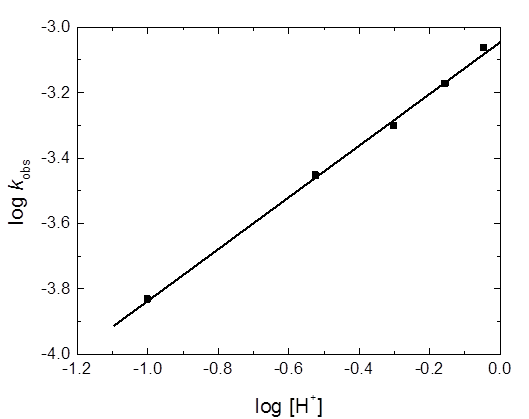Figure 3. A plot of log kobs versus log [H+] inthe oxidation of inositol by Ce(IV) in perchlorate medium. [INOS] = 6.0 x 10-3, [Ce(IV)] = 2.0 x 10-4 and I = 1.0  mol dm-3 at 25 oC.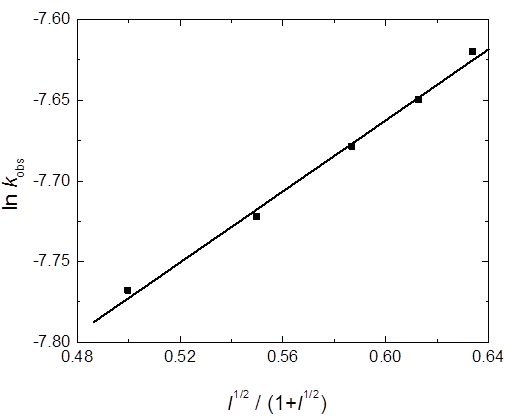Figure 4. Effect of ionic strength on kobs in the oxidation of inositol by Ce(IV) in perchlorate medium. [INOS] = 5.0 x 10-3 and [Ce(IV)] = 2.0 x 10-4mol dm-3 at 25 oC.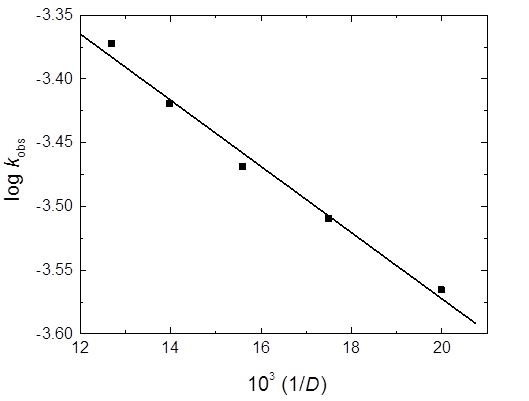Figure 5. Effect of dielectric constant of the reaction medium on kobs in the oxidation of inositol by Ce(IV) in perchlorate medium. [INOS] = 6.0 x 10-3, [Ce(IV)] = 2.0 x 10-4 and I = 1.0 mol dm-3 at 25 oC.

3.6. Effect of Initially Added Product

The effect of added cerium(III) product was studied in the concentration range 1.0 - 6.0 x 10-4mol dm-3 at fixed concentrations of the oxidant, reductant and acid. It was found that Ce(III) has no any significant effect on the oxidation rate of inositol.

3.7. Effect of Temperature

The oxidation rate was performed at five different temperatures in the range of 288 - 308 K, at constant concentrations of the reactants and other conditions being constant. The activation parameters of the second order rate constant (k2) are calculated using Arrhenius and Eyring plots and are listed in table 2.

Table 2. Activation parameters of the second order rate constants (k2) in the oxidation of inositol by Ce(IV) in perchlorate medium. [INOS] = 6.0 x 10-3, [Ce(IV)] = 2.0 x 10-4, [H+] = 0.5 and I = 1.0 mol dm-3.

 ΔS≠, J mol-1K-1 ΔH≠, kJ mol-1 ΔG≠298 ,kJ mol-1 Ea≠, kJ mol-1 -107.12 42.08 74.01 44.15

Experimental error ±4%

The reaction mixture to which a known quantity of acrylonitrile has been added initially was kept in inert atmosphere for 8 h. Upon diluting the reaction mixture with methanol, white precipitate has been formed, suggesting generation of free radical in the reaction. When the experiment was repeated in the absence of inositol under similar conditions, the test was negative. This indicates that the reaction was routed through free radical path.

4. Discussion

It has been reported [28-30] that active species of cerium (IV) in perchlorate medium were found to be either free monomeric species Ce4+, its hydrolyzed forms, Ce(OH)3+, Ce(OH)22+ or partially dimeric species (Ce–O–Ce)6+ and (HOCe–O–CeOH)4+. However, spectrophotometric studies showed  that the hydrated form, Ce4+, is the predominant species at [H+] 1.0 mol dm3 up to the concentration of 1.5 x 103 mol dm3 of cerium(IV), whereas the hydrolyzed forms and dimers are the more predominant at [H+] < 0.8 mol dm3. Therefore, under our experimental conditions and increasing the oxidation rate with the increase in [H+] suggests that Ce4+ may regarded as a more active form of Ce(IV) than its hydrolyzed form.

The reaction between inositol and Ce(IV) in perchlorate medium have a stoichiometry of 1:2, INOS : Ce(IV). The reaction exhibited first order dependence with respect to [Ce(IV)], less than unit order dependences with respect to both [H+] and [INOS]. The less than unit orders in [H+] suggests protonation of inositol in the first step to form protonated inositol as a more reactive species. On the other hand, the less than unit order with respect to inositol concentration suggests formation of an intermediate complex (C) between the kinetically active species of both inositol substrate and cerium(IV) oxidant prior to the rate-determining step. Complex formation was also proved kinetically by the non-zero intercept of the plot of 1/kobs versus 1/ [INOS]  as shown in figure 6. Also, increasing the oxidation rate upon increasing both ionic strength and dielectric constant of the reaction medium suggests that the reaction occurs between two similarly charged ions [33,34]. The rate is not considerably affected by Ce(III) suggesting that the rate-determining step should be irreversible as is generally the case for one electron oxidants  and the oxidation takes place throughout generation of free radicals as obtained experimentally.

Owing to the experimental results, a plausible reaction mechanism has been proposed for which all the observed orders in each constituent may be well accommodated. The proposed mechanism involves protonation of inositol in the first step followed by complexation of the protonated inositol (INOS+) with the kinetically active species of cerium(IV) oxidant (Ce4+) prior to the rate-determining step. Such complex decomposes to form a free radical intermediate derived from inositol substrate and Ce(III) ion. The free radical species further reacts with another species of the oxidant in a fast step to form the final oxidation product of inositol (inosose) as illustrated in the mechanistic Scheme 1.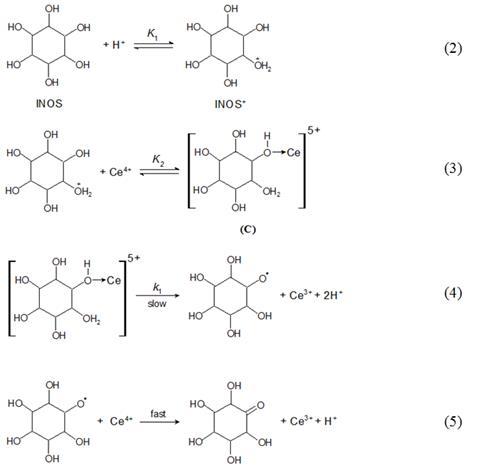Scheme 1. Mechanism of oxidation of inositol by Ce(IV) in perchlorate medium.

The suggested Scheme 1 lead to the following rate-law expression (see Appendix A),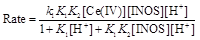(6)

The rate law (6) is consistent with all the observed orders with respect to different species.

Under pseudo-first order condition, the rate-law can be expressed by Eq. (7),

Rate =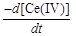= kobs [Ce(IV)]                  (7)

Comparing Eqs. (6) and (7),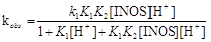(8)

and with rearrangement, the following equations are obtained,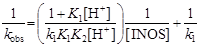(9)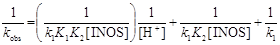(10)

Equations (9) and (10) requires that plots of 1/kobs versus 1/ [INOS] at constant [H+], and 1/kobs versus [H+] at constant [INOS] to be linear with positive intercepts and are found to be so as shown in figures 6 and 7, respectively. From the slopes and intercepts of such plots, the value of the rate constant of the rate-determining step (k1) and the equilibrium constants (K1 and K2) can be calculated.

Activation parameters listed in table 2 may be discussed as follows. The observed large negative values of entropy of activation, ΔS#, suggests that the reactants form an intermediate complex which are more ordered than the reactants, due to loss of degrees of freedom . Also, the obtained values of ΔS# are within the range of radical reactions. On the other hand, the positive values of both DH and DGindicate the endothermic formation of the intermediate complex and its non-spontaneity, respectively.

5. Conclusions

The oxidation of inositol by cerium(IV) in perchlorate medium was studied spectrophotometrically. The oxidation product of inositol was identified as inosose. The mechanistic scheme for the oxidation reaction has been proposed. The activation parameters of the second order rate constant were calculated and discussed.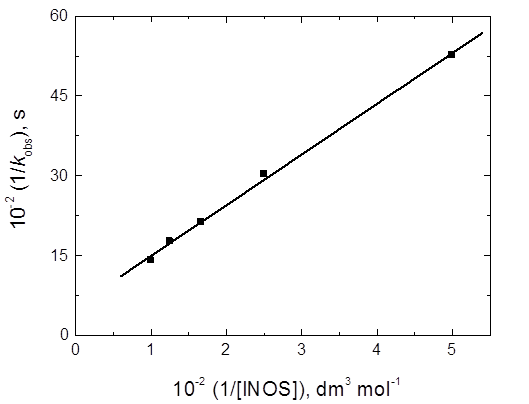Figure 6. Plot of 1/kobs against 1/ [INOS] inthe oxidation of inositol by Ce(IV) in perchlorate medium.[Ce(IV)] = 2.0 x 10-4, [H+] = 0.5 and I = 1.0 mol dm-3at 25 oC.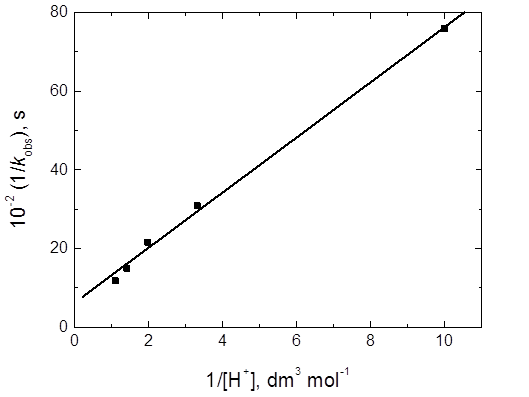Figure 7. Plot of 1/kobs against 1/ [H+]in the oxidation of inositol by Ce(IV) in perchlorate medium. [INOS] = 6.0 x 10-3,[Ce(IV)] = 2.0 x 10-4 and I = 1.0 mol dm-3at 25 oC.

Appendix A. Derivation of the rate-law expression:

According to the suggested mechanistic Scheme 1,

Rate == k1[C] (A1)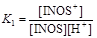,     [INOS+] = K1[INOS][H+] (A2)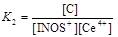,     [C] = K2[INOS+][Ce4+]       (A3)

Substituting Eq. (A2) into Eq. (A3) yields,

[C] = K1K2[INOS][Ce4+][H+](A4)

Also, substituting Eq. (A4) into Eq. (A1) gives,

Rate =k1K1K2 [INOS] [Ce4+] [H+](A5)     The total concentration of inositol is given by,

[INOS]T = [INOS]F + [INOS+] + [C] (A6)

where ‘T’ and ‘F’ refer to total and free concentrations.

Substituting Eq. (A2) and (A4) into Eq. (A6) gives,

[INOS]T = [INOS]F + K1 [INOS] [H+] +

K1K2 [INOS] [Ce4+] [H+] (A7)

[INOS]T = [INOS]F(1 + K1[H+] + K1K2[Ce4+][H+]) (A8)

Therefore,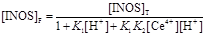(A9)

Also, the total concentration of cerium (IV)equals to,

[Ce(IV)]T = [Ce4+]F + [C](A10)

[Ce(IV)]T = [Ce4+]F+ K1K2[INOS][Ce4+][H+](A11)

[Ce(IV)]T = [Ce4+]F(1+ K1K2[INOS][H+])(A12)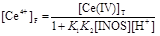(A13)

Due to high [H+],       [H+]T = [H+](A14)

Substituting Eqs. (A9), (A13) and (A14) into Eq. (A5) (and omitting ‘T’ and ‘F’ subscripts) gives,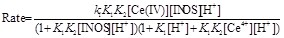(15)

Owing to low [Ce4+], the last denominator term in Eq. (A15) is neglected. Therefore,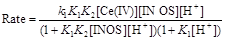(A16)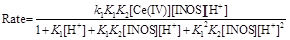(A17)

Due to low [INOS], the denominator term, K12K2 [INOS] [H+]2, in Eq. (A17) can be neglected,

Therefore,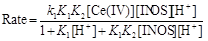(A18)

Under pseudo-first order condition, the rate-law can be expressed by Eq. (A15),

Rate =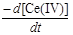= kobs[Ce(IV)](A19)

Comparing Eqs. (A18) and (A19),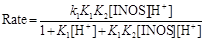(A20)

and with rearrangement, the following equations are obtained,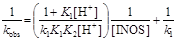(A21)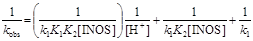(A22)

References

1. Michell RH (2007) Evolution of the diverse biological roles of inositols. BiochemSoc Symp. 74: 223-246.
2. Shen X, Xiao H, Ranallo R, Wu W H and Wu C (2003) Modulation of ATP-dependent chromatin-remodeling complexes by inositol polyphosphates. Science 299:112-114.
3. Rapiejko PJ, Northup JK, Evans T, Brown JE, Malbon CC (1986) G proteins of fat cells role in hormonal regulation of intracellular inositol 1,4,5-trisphosphate. Biochem. J. 240: 35-40.
4. Larner J (2002) D-chiro-inositol –iIts functional role in insulin action and its deficit in insulin resistance. Int. J. Exp. Diabetes Res. 3:47-60.
5. Kumar YL, Nadh RV, Radhakrisinami PS (2012) Kinetics of oxidation of myo-inositol by potassium periodate in alkaline medium.Asian J.Chem. 24:5869-5872.
6. Nayak GT, Hadmani CC, Harihar AL (2015) Kinetic and mechanistic investigations of oxidation of myo-inositol by diperiodatocuprate (III) in aqueous alkaline medium. Chem. Sci. Trans. 4:199-207.
7. Nayak GT, Hadmani CC, Harihar AL (2014) Oxidation of myo-inositol by alkaline permanganate and the effect of alkali metal ion catalysts: kinetic and mechanistic approach. Know Res. 1:33-39.
8. Kumar A, Rain M (1974) Mechanism of oxidation of cyclohexanehexol (inositol) by quinquevalent vanadium (pages. Int J ChemKinet. 6:15-28.
9. Santoro M, Caffaratti E, Salas-Peregrin GM, Korecz L, Rockenbauer A, Sala LF, Signorella F (2007)Kinetics and mechanism of the chromic oxidation of myo-inositol. Polyhedron 26:169–177.
10. Hassan RM, Alaraifi A, Fawzy A, Zaafarany IA, Khairou KS, Ikeda Y and Takagi HD (2010) Acid-catalyzed oxidation of some sulfated polysaccharides. Kinetics and mechanism of oxidation of kappa-carrageenan by cerium (IV) in aqueous perchlorate solutions. J. Mol. Cat. A, 332:138–144.
11. Fawzy A (2016) Oxidation of alginate and pectate biopolymers by cerium (IV) in perchloric and sulfuric acid solutions: A comparative kinetic and mechanistic study. Carbohydr. Polym. 138: 356-364.
12. Fawzy A (2016)Kinetic and mechanistic aspects of oxidation of aminotriazoleformamidine by cerium (IV) in aqueous perchloric and sulfuric acid solutions: a comparative study.J. Solution Chem. 45:46-264.
13. Fawzy A, Hassan R M, Althagafi I, Morad M (2016) Cerium (IV) oxidation of sulfated polysaccharides in aqueous perchlorate solutions. A Kinetic and mechanistic approach. Adv. Mater. Lett. 7: 122-128.
14. Fawzy A, Zaafarany I A, Tirkistani F A, Al-Benayan A and Aljiffrey F A (2016) Kinetics and mechanistic study of oxidation of pyridine derivative by cerium (IV) in aqueous perchloric acid, Am. J. Phys. Chem. 5:10-16.
15. Richardson W H (1965) Oxidation of organic chemistry, In K B Wiberg (ed.) (Academic Press: London) p. 244.
16. Adari KK, Nowduri A, Parvataneni V (2008) Kinetics and mechanism of oxidation of L-cystine by cerium (IV) in sulphuric acid medium. ActaChim. Slov. 55:425–429.
17. Sumathi T, Shanmugasundaram P and Chandramohan G (2013) A kinetic and mechanistic study on the silver (I) catalyzed oxidation of L-Serine by cerium (IV) in sulfuric acid medium. J. Saudi Chem. Soc. 17:227-233.
18. Thabaj KA, Chimatadar SA, Nandibewoor ST (2006) Mechanistic study of oxidation of palladium (II) by cerium (IV) in aqueous acid. Transition Met. Chem., 31:186-193.
19. Datt N, Nagori RR, Mehrotra RN (1986) Kinetics and mechanisms of oxidations by metal ions. Part VI. Oxidation of α-hydroxy acids by cerium (IV) in aqueous nitric acid. Can. J. Chem. 64: 19-23.
20. McCurdy, Jr WH, Guilbault GG (1960) Catalysts for cerium (IV) oxidimetry: determination of mixtures of mercury (I) and mercury (II). Anal. Chem. 32:647-650.
21. Mishra SK, Gupta YK (1970) Kinetics of oxidation of antimony (III) by cerium (IV) in media containing perchloric acid. J. Chem. Soc. A, 260-264.
22. Yadav MB, Derva V, Rani A (2009) Kinetics and mechanism of uncatalyzed and silver (I) catalyzed oxidation of lysine by cerium (IV) in acid perchlorate medium. J. Indian Chem. Soc. 86: 600-604
23. Mathur S, Yadav MB, Devra V (2013) Kinetics and mechanism of uncatalyzed and Ag (I) catalyzed oxidation of hydroxylysine by cerium (IV) in acid medium.J. Phys. Chem. Biophys. 3:5-12.
24. Mathur S, Yadav MB, Devra V (2015) Kinetics and mechanism of uncatalyzed and Ag (I) catalyzed oxidation of serine by cerium (IV) in acid medium. Int. J. Res. Phys. Chem. 5:1-6.
25. Hardwick TJ, Robertson E (1951) Ionic species in ceric perchlorate solutions. Can. J. Chem., 29: 818-828
26. Furniss BS, Hannaford AJ, Smith WG, Tatchell AR (2004) In Vogel’s textbook of practical organic chemistry, 5th (ed.) (Pearson Education Ltd)
27. Vogel A I (1973) In Text book of practical organic chemistry, 3rd (ed.) (London: Longman) p. 332
28. Feigl F (1975) Spot tests in organic analysis, p. 195, Elsevier, New York.
29. Sherill MS, King CB, Spooner RC (1943) The oxidation potential of cerous-ceric perchlorates. J. Am. Chem. Soc. 65:170-179.
30. Heidt LJ, Smith ME (1948) Quantum yields of the photochemical reduction of ceric ions by water and evidence for the dimerization of ceric ions. J. Am. Chem. Soc., 70: 2476-2481
31. King EL, Pandow ML (1952) The spectra of cerium (IV) in perchloric acid. Evidence for polymeric species. J. Am. Chem. Soc. 74:1966-1969
32. Offner HG, Skoog DA (1966) Hydrolysis constant of quadrivalent cerium from spectrometric measurements. Anal. Chem. 38:1520-1521
33. Michaelis L, Menten ML (1913) The kinetics of invertase action. Biochem. Z., 49:333–369
34. Frost AA, Person RG (1973) Kinetics and mechanism, p. 147, Wiley Eastern, New Delhi
35. Amis ES (1966) Solvent effect on reaction rates and mechanism, p. 28, Academic Press, New York
36. Weissberger A (1974) In Investigation of rates and mechanism of reactions in techniques of chemistry, (New York: John Wiley & Sons), p. 421.

 Contents 1. 2. 2.1. 2.2. 3. 3.1. 3.2. 3.3. 3.4. 3.5. 3.6. 3.7. 3.8. 4. 5.
Article ToolsAbstractPDF(304K)